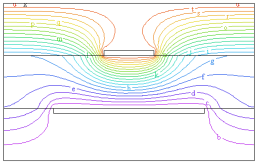﻿ Sample Problems > Usage > 3D_domains > 3d_extrusion_spec

# 3d_extrusion_spec

Navigation:  Sample Problems > Usage > 3D_domains >

# 3d_extrusion_spec{ 3D_EXTRUSION_SPEC.PDE

This descriptor is a demonstration of the grammar of 3D extrusions.

It is a completion of the 3D specification example shown in

It describes a strip capacitor fabricated as a sandwich of

air | metal | glass | metal | air.

Click "Controls->Domain Review" to watch the domain construction process.

See the sample problem "3D_Capacitor" for a somewhat more complicated

and interesting version.

}

 TITLE '3D Extrusion Spec' SELECT regrid=off { for quicker solution }   COORDINATES CARTESIAN3   DEFINITIONS  Kdiel= 6  Kmetal=1e6  Kair=1  K = Kair     { default to Kair }  V0 = 0  V1 = 1   VARIABLES V   EQUATIONS  V:  DIV(K*GRAD(V)) = 0EXTRUSION

SURFACE       "Bottom"                        Z=0

LAYER       "Bottom Air"

SURFACE       "Bottom Air - Metal"            Z=0.9

LAYER       "Bottom Metal"

SURFACE       "Bottom Metal - Dielectric"     Z=1

LAYER       "Dielectric"

SURFACE       "Top Metal - Dielectric"        Z=2

LAYER       "Top Metal"

SURFACE       "Top Metal - Air"               Z=2.1

LAYER       "Top Air"

SURFACE       "Top"                           Z=3

BOUNDARIES

SURFACE "Bottom" VALUE(V)=0

SURFACE "Top" VALUE(V)=1

REGION 1                         { this is the outer boundary of the system }

LAYER "Dielectric" K = Kdiel { all other layers default to Kair }

START(0,0)

LINE TO (5,0) TO (5,5) TO(0,5) to close

LIMITED REGION 2     { this region exists only in the "bottom metal" layer,

and describes the larger plate }

LAYER "Bottom Metal" K = Kmetal

START(1,0)

LAYER "Bottom Metal" VALUE(V)=V0

LINE TO (4,0)

LAYER "Bottom Metal" NATURAL(V)=0

LINE TO (4,5) TO (1,5) to close

LIMITED REGION 3     { this region exists only in layer "Top Metal",

and describes the smaller plate }

LAYER "Top Metal" K = Kmetal

START(2,0)

LINE TO (3,0) TO (3,5)

LAYER "Top Metal" VALUE(V)=V1

LINE TO (2,5)

LAYER "Top Metal" NATURAL(V)=0

LINE to close

SELECT painted

PLOTS

CONTOUR(V) ON X=2.5 as "V on X-cut"

CONTOUR(V) ON Y=2.5 as "V on Y-cut"

CONTOUR(V) ON Z=1.5 as "V on Z-cut"

GRID(x,z) ON Y=2.5 paintregions nolines as "Region Map"

GRID(x,z) ON Y=2.5 paintmaterials nolines as "Material Map"

GRID(x,y,z) ON LAYER 2 ON REGION 2 as "Bottom Plate"

GRID(x,y,z) ON "Top Metal" ON REGION 3 as "Top Plate"

END